Name:    2nd Grade Numbers in Operations in Base Ten- Understand place value, Standard 1, Pre Test 1 Understand that the three digits of a three-digit number represent amounts of hundreds, tens, and ones; e.g., 706 equals 7 hundreds, 0 tens, and 6 ones.

Multiple Choice
Identify the choice that best completes the statement or answers the question.

1.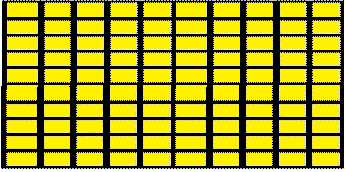What is the sum of all the colored squares?
 a. 100 c. 80 b. 10 d. 90

2.

In the number 256, what is the value of the 5?
 a. 5 c. 10 b. 10 d. 50

3.

What is the total of the colored squares in the array?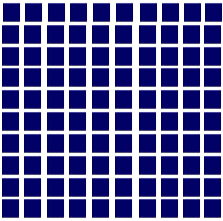a. 10 c. 1000 b. 90 d. 100

4.

In the number 712, what is the place value of the 2.
 a. 2 c. 200 b. 20 d. 10

5.

In the number 347, what is the value of the 3?
 a. 3 c. 30 b. 14 d. 300

Numeric Response

6.

THE NEXT QUESTIONS ARE ALL ANSWERED WITH JUST A NUMBER.
In the number below, which number is in the tens place?
987

7.

In the number below, which number is in the ones place?
123

8.

In the number below, which number is in the hundreds place?
498

9.

In the number below, which number is in the hundreds place?
3100

10.

In the number 700, how many tens are there?

11.

In the number 100, how many hundreds are there?

12.

In the number 800, how many ones are there?

13.

In the number 57241, which number holds the hundreds place value?

14.

What number does this diagram represent?

100’s               10’s            1’s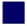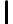15.

What number does this diagram represent?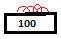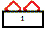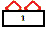16.

If Marty has six bags of candy that hold 100 pieces each, five cups that hold 10 pieces each and three pieces by themselves, how many total pieces of candy does he have?